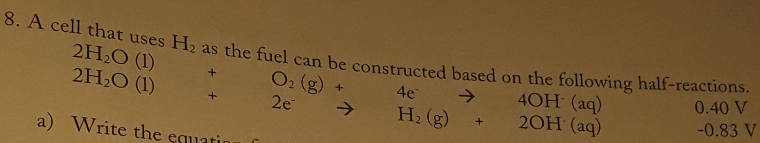# 8. A cell that uses H2 as the fuel can be constructed based on the following half-reactions. 2H2O(I) + O2(g) + 4e^- → 4OH^-(aq) 0.40 V 2H2O(I) + 2e^- → H2(g) + 2OH^-(aq) -0.83 V b) Calculate the standard potential for the cell at 298 K.